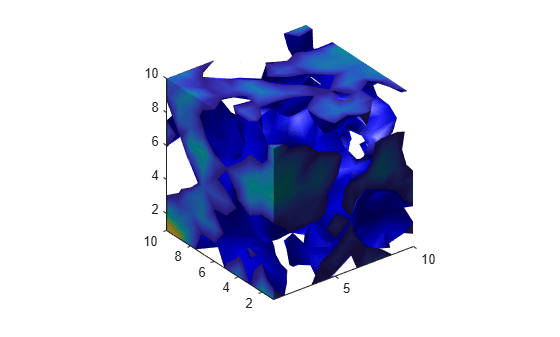Main Content

# smooth3

Smooth 3-D data

## Description

`W = smooth3(V)` smooths the input data `V` and returns the smoothed data in `W`.

`W = smooth3(V,'filter')` `filter` determines the convolution kernel and can be one of these values:

• `'gaussian'`

• `'box'` (default)

`W = smooth3(V,'filter',size)` sets the size of the convolution kernel (default is `[3 3 3]`). If `size` is scalar, then `size` is interpreted as [`size`, `size`, `size`].

`W = smooth3(V,'filter',size,sd)` sets an attribute of the convolution kernel. When `filter` is `gaussian`, `sd` is the standard deviation (default is .65).

## Examples

collapse all

Create a 10-by-10-by-10 array of random data and smooth it.

```data = rand(10,10,10); data = smooth3(data,'box',5);```

Display the data as an isosurface with end caps.

```patch(isocaps(data,.5),... 'FaceColor','interp','EdgeColor','none'); p1 = patch(isosurface(data,.5),... 'FaceColor','blue','EdgeColor','none'); isonormals(data,p1); view(3); axis vis3d tight camlight left lighting gouraud```## See Also

Introduced before R2006a

Download ebook